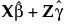Publication date: 11/29/2021

##Conditional Profilers

The conditional model profiler plots are based on conditional predicted values. These are the predicted values obtained if you select Save Columns > Conditional Prediction Formula.

Denote the linear mixed model by E[Y|γ] = Xβ + Zγ. Here β is the vector of fixed effect coefficients and γ is the vector of random effect coefficients. The conditional predictions are the predictions obtained from the model given by.

Four types of profilers are provided:

Conditional Profiler

Conditional Contour Profiler

Conditional Mixture Profiler

Conditional Surface Profiler

Options that are appropriate for the model that you are fitting are enabled. See Figure 8.21 for an example of a Profiler. See Figure 8.42 for an example of a Surface Profiler. For more information about the profiler, see Surface Plot in Profilers.